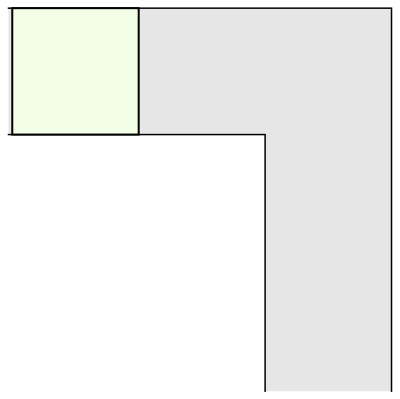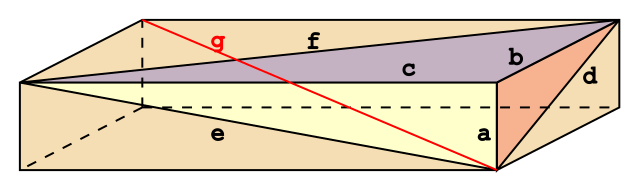## MATHS BITE: Sierpinski Number

A Sierpinski number is an odd natural number k such thatis not prime for all natural numbers n. In 1960, Sierpinski proved that there are infinitely many odd integers k with this property, but failed to give an example. Numbers in such a set with odd k and k < 2^n are called Proth numbers.

78557, 271129, 271577, 322523, 327739, 482719, 575041, 603713, 903983, 934909,….

-List of some known Sierpinski Numbers

### Sierpinski Problem

The Sierpinski problem asks what the smallest Sierpinski number is. In 1967, Sierpinski and Selfridge conjectured that 78557 is the smallest Sierpinski number. To show this is true, we need to show that all the odd numbers smaller than 78557 are not Sierpinski numbers, i.e. for every odd k below 78557 there is a positive interger n such thatis prime. There are only five numbers which have not been eliminated:

k = 21181, 22699, 24737, 55459, and 67607

### Numberphile Video

Find out more here. M x

## Knapsack Problem

The knapsack problem is a problem in combinatorial optimisation.

Imagine you are going to visit your friends for whom you have bought lots of presents. So many, in fact, that you can’t fit them all in your luggage meaning you must leave some behind. If we decide to pack a combination of presents with the highest value but that doesn’t exceed the weight limit imposed by airline, how can we find such a combinations? This is the Knapsack Problem.

We could solve this by trying all the possible combinations, calculating their value and weight and then picking one that is below the weight limit but maximises the value. Whilst this is okay when we are dealing with a small number of items, it is simply unfeasible when the number of items is large as the number of combinations is too big. So, is there an algorithm which can work for any number of items that doesn’t take too long?

Computer scientists have a way of measuring the complexity of a problem by how fast the time taken grows as the size of the input increases. Polynomial growth, i.e. if the size of the input is n then the time taken grows by a factor of n^k, describes and ‘easy problem’; the growth is nowhere near as “explosive” as  exponential growth, e.g. 2^n.

Whether or not a polynomial time algorithm exists to solve the knapsack problem is unknown, meaning it is in a class of problems called NP-Hard Problems.

Complexity Classes:

• Class P: problems that can be solved in polynomial time
• Class NP: a potential solution can be checked in polynomial time
• NP-Complete: within the NP class but particularly hard
• NP-Hard: as least as hard as the NP class.

It is possible that the NP class = P class, though this is still unknown (and is one of the Millennium Prize Problems), hence the two diagrams above.

M x

P.S. My posts may be more sporadic in the next month because I’m in exam term!

## Unsolved Problems: Part II

This is a continuation of my post from last Thursday on simple unsolved problems in mathematics.

### Inscribed Square Problem

The inscribed square problem is an unsolved problem in geometry, proposed by German mathematician Otto Toeplitz in 1911. It asks whether it is possible to draw a square inside a closed loop so that all four corners of the square are contained in this closed curve.

Some early positive results were obtained by Arnold Emch and Lev Schnirelmann, but the general case still remains open. An example of a positive result is shown below:This has already been solved for other shapes, such as triangle and rectangles, but the case with squares is proving trickier to solve.

### Happy Ending Problem

The Happy Ending problem, so named by Paul Erdős because it led to the marriage of Esther Klein and George Szekeres, is the following statement:

“Theorem: any set of five points in the plane in general position has a subset of four points that form the vertices of a convex quadrilateral.”

In other words, if you make five dots on a piece of paper, you should always be able to connect four of them to create a convex quadrilateral (i.e. a shape with four sides where all the corners are less than 180 degrees) regardless of the position of these dots.

Although the result for a four sided shape is known (and in fact it is known for a pentagon – 9 dots are needed – and a hexagon – 17 dots are needed), it is still unknown how many dots is needed to create a shape with a greater number of sides.

Mathematicians speculate that the equation which determines the number of dots M can be related to the number of sides in the shape N by the following equation:

M=1+2N-2

However, it has only yet been proved that this will give a number AT LEAST as big as the answer.

Hope you enjoyed this mini-series! Let me know if you want me to continue it. M x

## Unsolved Problems: Part I

There are many unsolved problems in mathematics. (Most too complicated for me to even understand, let alone explain in a blog post!) However, this week I am going to talk about 4 simple unsolved problems in mathematics in a two part series.

### Moving Sofa Problem

In 1966, mathematician Leo Moser posed the following mathematical problem:

“What is the shape of largest area in the plane that can be moved around a right-angled corner in a two-dimensional hallway of width 1?”

In other words, this problem asks what is the rigid two-dimensional shape with largest area A that can be moved through and L shaped region with unit width. It is an idealisation of real-life furniture moving problem, and thus the area A obtained is referred to as the sofa constant.

Let us look at few different examples.

Unit Square:The area of this square is one, and therefore very low. It is easy to find a better shape.

Semi-Circle:The area of this shape is greater than that of the unit square: it is ᴨ/2. Additionally, it is more interesting because it order for it to move around the corner, it must rotate, whereas the unit square merely translates.

Now, can we find a shape that can rotate and translate around the corner in order for the area to be maximised? Mathematician John Hammersley noticed that cutting a semicircle into two and filling the gap with a rectangular block would create a shape, which could be moved around the corner if only a smaller semicircular hole is also removed from the rectangular block. In doing so, he found a shape with A = 2/ᴨ+ᴨ/2 that can move around the L-shape.Hammersley thought that this construction was optimal, but it turned out that it was not. In 1992, Joseph Gerver found a better shape with a slightly bigger area of around 2.2195.Furthermore, Hammersley found an upper bound on the sofa constant, showing that it is at most, however the shape corresponding to this value has not been found.

### Perfect Cuboid Problem

This problem is an extension of the Pythagorean Theorem – A2 + B2 = C– into 3 dimensions.In three dimensions there are four numbers, which correspond to A, B, C and G in the image above; A, B, C are the dimensions of the cuboid, and G is the diagonal running from one of the top corners to the opposite bottom corner. But, there are three more diagonals on the three surfaces, labelled D, E and F, which raises the question of whether there can be a box where all seven of these lengths are integers.

In other words, the goal is to find a cuboid such that A2 + B2 + C2 = G2 and where all 7 lengths are integers. Mathematicians have still not been able to find an example of such a cuboid, but they have also not been able to show that it is not possible. So, it remains unsolved.

Stay tuned for part 2 on Monday! M x